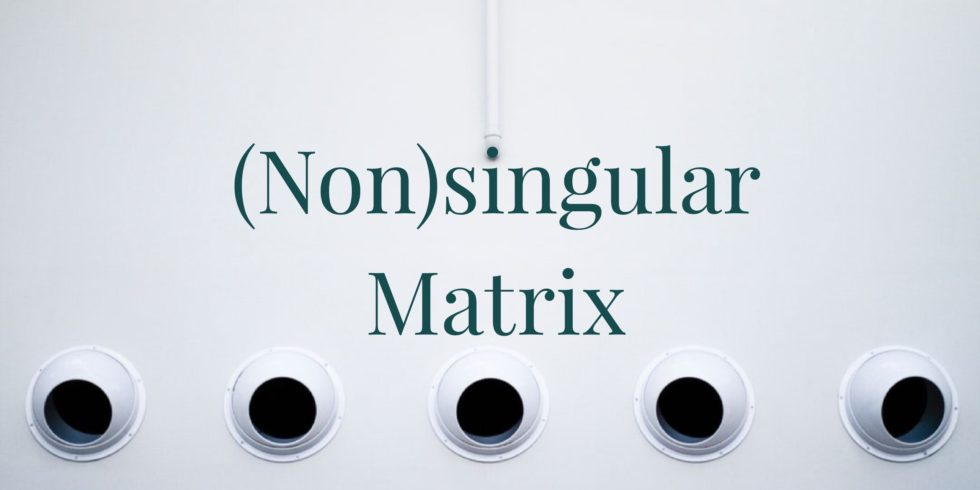# Show that the Given 2 by 2 Matrix is Singular## Problem 655

Consider the matrix $M = \begin{bmatrix} 1 & 4 \\ 3 & 12 \end{bmatrix}$.

(a) Show that $M$ is singular.

(b) Find a non-zero vector $\mathbf{v}$ such that $M \mathbf{v} = \mathbf{0}$, where $\mathbf{0}$ is the $2$-dimensional zero vector.Add to solve later

## Solution.

### (a) Show that $M$ is singular.

To see that $M$ is singular, we will row-reduce the augmented matrix:
$\left[\begin{array}{rr|r} 1 & 4 & 0 \\ 3 & 12 & 0 \end{array} \right] \xrightarrow{R_2 – 3 R_1} \left[\begin{array}{rr|r} 1 & 4 & 0 \\ 0 & 0 & 0 \end{array} \right].$ The row-reduced matrix has a row of all $0$’s, and hence is singular.

### (b) Find a non-zero vector $\mathbf{v}$ such that $M \mathbf{v} = \mathbf{0}$.

We see that the vector $\mathbf{v} = \begin{bmatrix} v_1 \\ v_2 \end{bmatrix}$ must satisfy the equation $v_1 + 4 v_2 = 0$.

There are many solutions. One solution, for example, is found by setting $v_1 = 1$ and solve $v_2 = – \frac{1}{4}$.Add to solve later

### More from my site

#### You may also like...

This site uses Akismet to reduce spam. Learn how your comment data is processed.

###### More in Linear Algebra##### Column Vectors of an Upper Triangular Matrix with Nonzero Diagonal Entries are Linearly Independent

Suppose $M$ is an $n \times n$ upper-triangular matrix. If the diagonal entries of $M$ are all non-zero, then prove...

Close